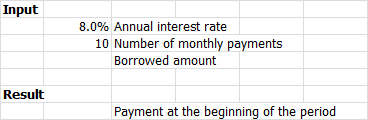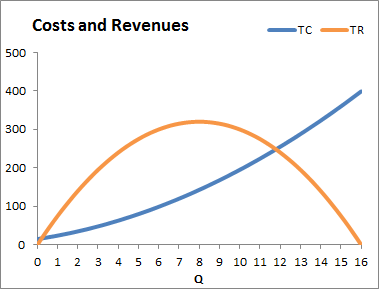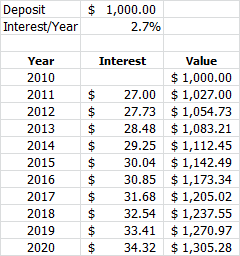# 14  Goal Seek

OBJECTIVES

• Goal Seeking Method.
• Application field for Goal Seeking.
• Tips using Goal Seek.

A formula is made up of one or more variables. Usually you want to know what the result of the formula is for certain values of the variables. Sometimes the reverse also occurs. For a certain outcome you want to know which values the variables should have.

When the outcome of a formula only depends on one variable, you can use the method Goal Seek. This method changes the value of the variable until the formula has the desired result.

When the outcome of a formula depends on several variables, you should use Excels Solver.

Dialog box Goal Seek

Goal Seeking can be accessed via tab Data > What-If Analysis (group Forecast) > Goal Seek.

In the dialog box you need to specify three values:

Set cell
The address of the cell that contains the formula for which you want a certain result.
To value
The desired outcome of the formula, in other words the goal.
By changing cell
The address of the cell whose value must be changed to achieve the target. This is the cell for the variable.

## 14.1 Break-even coffee stand

Suppose you want to run a coffee stand in a soccer stadium. The monthly costs for rent, staff and miscellaneous are known. You also know the costs and sales price for a cup of coffee. A calculation model has been made for the calculation of the monthly result, see Figure 14.2, depending on the number of cups of coffee sold.

Information need
How many cups of coffee do you need to sell per month to break even?

In this simple example, you can easily calculate the answer by hand. However, Goal Seeking is very suitable for such issues.

Task 14.1 File: Stadium_Coffee.xlsx

1. Open the file.

2. Choose tab Data > What-If Analysis (group Forecast) > Goal Seek.

3. Specify the values for Goal Seek.

• Set cell: The cell with the month result, B18.
• To value: Zero for break-even.
• By changing cell: The cell with the number of cups of coffee to be sold, B16.
1. Click OK.

Excel starts calculating and finds the value 562.5. Because you don’t sell half cups of coffee you will need to round the answer to 563.

## 14.2 Tips Goal Seek

It is possible that the result function is structured in such a way that the desired answer does not exist. It is also possible that the answer exists, but that Excel cannot find it. In that case, a dialog box will appear indicating this.

If the solution exists, but Excel can not find it, then check the following possible solutions:

1. Check in the model that the result cell depends on the changing cell. The result cell must always contain a formula or a function.
2. Check that the cell to be modified contains only a value and no formula or function.
3. Choose different initial values for the cell to be modified.
4. Increase the maximum number of iterations (default is 100) via File > Options > Formulas, see Figure 14.4.

## 14.3 Exercises

Freelancer (goal001)

Suppose you want to work as a freelancer and you have the intention to do this only if you can earn at least $5000,- per month. You get a commission of 7.8% for each project. 1. Create a model in a worksheet in which you can enter the total sales and the percentage of commission. Calculate the commission. 2. Determine through Goal Seek the total sales that must be made to get a commission of$5000.

Total sales $64,102.56 Loan payment (goal002) Using the PMT function you can calculate the periodic payment of a loan based on constant payments and a constant interest rate. The following illustration is an example of such a calculation. The payment is done at the beginning of each term and after the last term, the loan is fully paid off.1. Create this model in a worksheet. 2. Determine with Goal Seek how much you can borrow if you can pay up to$750,-per month.

Because payment is a cash outflow, the outcome is seen as a negative number.

Borrowed amount $7280. Break-even point (goal003) An entrepreneur has to deal with costs and revenues that depend on the production quantity Q. The relationship between the total cost (TC), the total revenues (TR) and the production quantity Q is as follows: $$\mathrm{TC = Q^2 + 8Q + 15}$$ and $$\mathrm{TR = -5Q^2 + 80Q}$$ In the following chart, TC and TR are plotted as a function of Q.1. Draw this chart. 2. Determine the break-even point. This is the amount of Q for which the costs and the revenues are equal. The chart shows that there are two of these points. Determine both solutions using Goal Seek and give the answers in 4 decimal places. Solution 1: Q=0,2120 and TC=TR=16,74 Solution 2: Q=11,7879 and TC=TR=248,26 Savings amount (goal004) On 1 January 2010, an amount of$ 1000 is deposited into a savings account. The interest is 2.7% annually and this is added to the savings account. In the following table, you see the growth of the amount on the savings account for the first ten years.1. Create the model in a worksheet.
2. Determine with Goal Seek how much the deposit should be at the beginning to get $2500 on the savings account after 10 years. Deposit$ 1915,29.